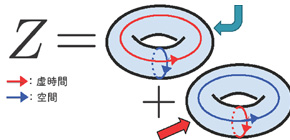## Successful calculation of the exact partition function of the three dimensional quantum gravity theory

### The results of an origin theory to understand the creation of the universe, from the microscopic world to its far reaches

Oct 23, 2015

Graduate School of Science, Osaka University -- IIZUKA Norihiro (Assistant Professor), TANAKA Akinori (then graduate school student, Osaka University; currently Special Postdoctoral Researcher, RIKEN)
Yukawa Institute for Theoretical Physics, Kyoto University -- TERASHIMA Seiji (Assistant Professor)

A group of researchers from Osaka University and Kyoto University succeeded in calculating the exact partition function of the three-dimensional (2 spatial and 1 temporal) quantum gravity theory when a cosmological constant is negative under a certain equivalence, a world first. Quantum gravity theory is a theory integrating the two main pillars of modern physics: quantum mechanics and Einstein’s general theory of relativity.

Quantum mechanics calculates the path particles, such as electrons, take. Path integral, the basic principle of quantum mechanics, was difficult to calculate with conventional methods; therefore, researchers could only rely on approximate methods. On the other hand, three-dimensional Euclidean pure gravity theory is known to be equivalent to Chern-Simons theory, classically.

This group succeeded in exactly calculating path integral (a key point in quantum theory) in Chern-Simons theory by using the recently developed localization technique.  As Chern-Simons theory is thought to be formulated in terms of three-dimensional Euclidean pure gravity, it can be said that this group performed exact calculation of three-dimensional Euclidean pure gravity theory.

In order to understand questions such as “How did the universe begin before the Big Bang?” and “What is happening at the center of a black hole?” quantum gravity theory beyond the framework of a standard modern physics theory is necessary. Quantum gravity theory is a key to understanding the creation of the universe. This group’s research results are a step toward understanding quantum gravity theory currently being developed. The results will serve as a stepping stone to further understanding of quantum gravity theory, quantum field theory, and gauge/gravity duality as well.

Abstract

Three dimensional Euclidean pure gravity with a negative cosmological constant can be formulated in terms of the Chern-Simons theory, classically. This theory can be written in a supersymmetric way by introducing auxiliary gauginos and scalars. We calculate the exact partition function of this Chern-Simons theory by using the localization technique. Thus, we obtain the quantum gravity partition function, assuming that it can be obtained non-perturbatively by summing over partition
functions of the Chern-Simons theory on topologically different manifolds. The resultant partition function is modular invariant, and in the case in which the central charge is expected to be 24, it is the J-function, predicted by Witten.Figure 1. To calculate the partition function Z of the three-dimensional quantum gravity, it is necessary to perform a path-integral of spacetimes. In this research, the exact partition function of the three-dimensional quantum gravity was calculated with use of localization, by identifying the path-"integral" of the spacetimes with a "summation" of exact solutions of Chern-Simons theory on various spacetimes.